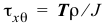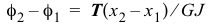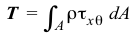# 5.1 Torsion of Circular Shafts

In the questions below, select the correct answer.

NameEmail
1) Torsional shear strain varies linearly across a homogeneous cross section.
2) Torsional shear strain is a maximum at the outermost radius for a homogeneous and a nonhomogeneous cross section.
3) Torsional shear stress is a maximum at the outermost radius for a homogeneous and a nonhomogeneous cross section.
4) The formulacan be used to find the shear stress on a cross section of a tapered shaft.
5) The formulacan be used to find the relative rotation of a segment of a tapered shaft.
6) The formulacan be used to find the shear stress on a cross section of a shaft subjected to distributed torques.
7) The formulacan be used to find the relative rotation of a segment of a shaft subjected to distributed torques.
8) The equationcannot be used for nonlinear materials.
9) The equationcan be used for a nonhomogeneous cross section.
10) Internal torques jump by the value of the concentrated external torque at a section.